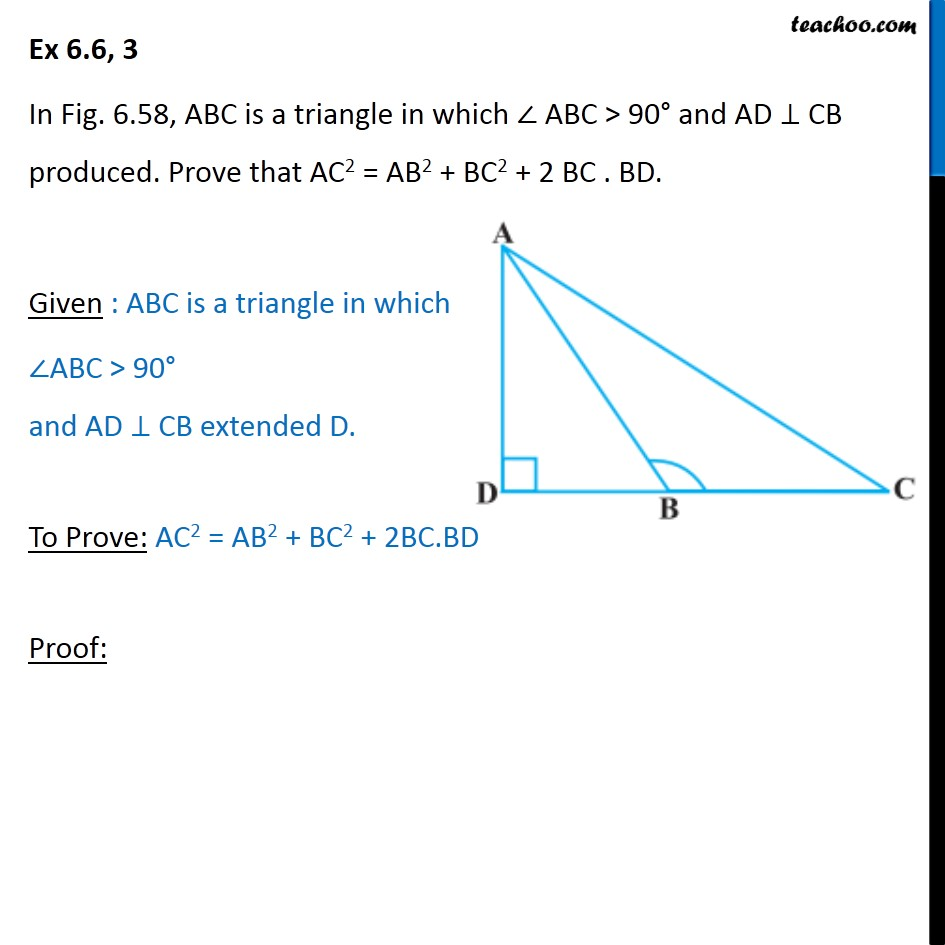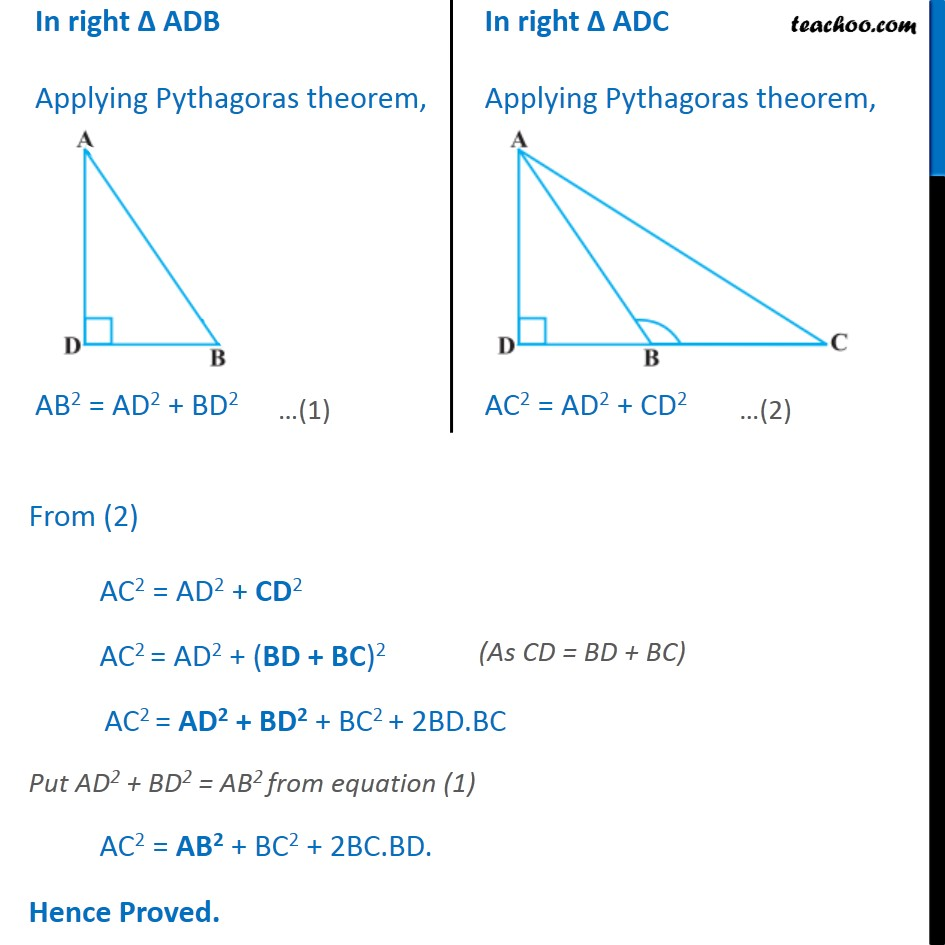Ex 6.6 (Optional)

Chapter 6 Class 10 Triangles
Serial order wiseMaths Crash Course - Live lectures + all videos + Real time Doubt solving!

### Transcript

Ex 6.6, 3 In Fig. 6.58, ABC is a triangle in which ABC > 90 and AD CB produced. Prove that AC2 = AB2 + BC2 + 2 BC . BD. Given : ABC is a triangle in which ABC > 90 and AD CB extended D. To Prove: AC2 = AB2 + BC2 + 2BC.BD Proof: In right ADB Applying Pythagoras theorem, AB2 = AD2 + BD2 In right ADC Applying Pythagoras theorem, AC2 = AD2 + CD2 From (2) AC2 = AD2 + CD2 AC2 = AD2 + (BD + BC)2 AC2 = AD2 + BD2 + BC2 + 2BD.BC Put AD2 + BD2 = AB2 from equation (1) AC2 = AB2 + BC2 + 2BC.BD. Hence Proved.